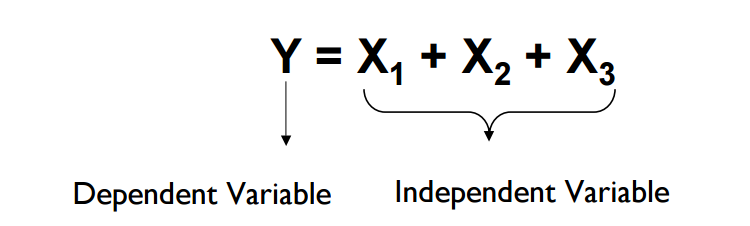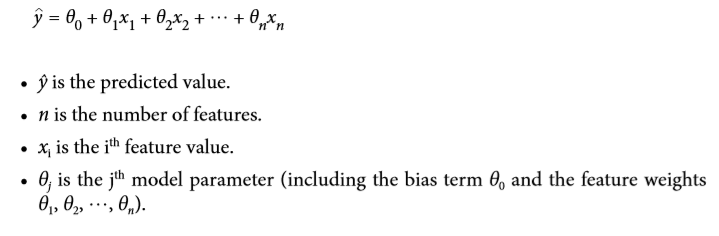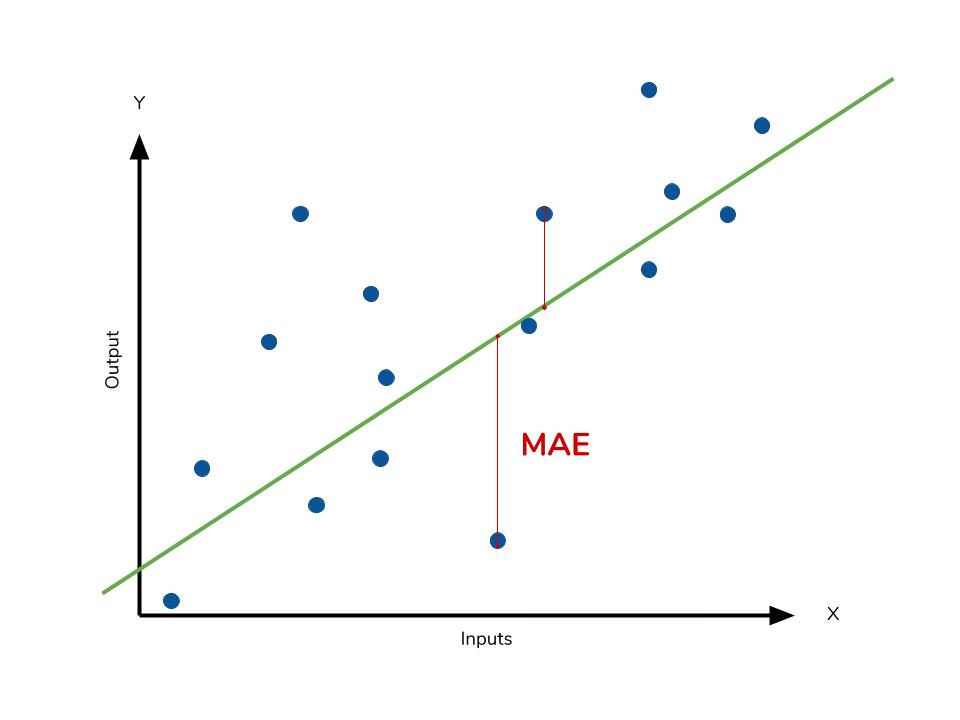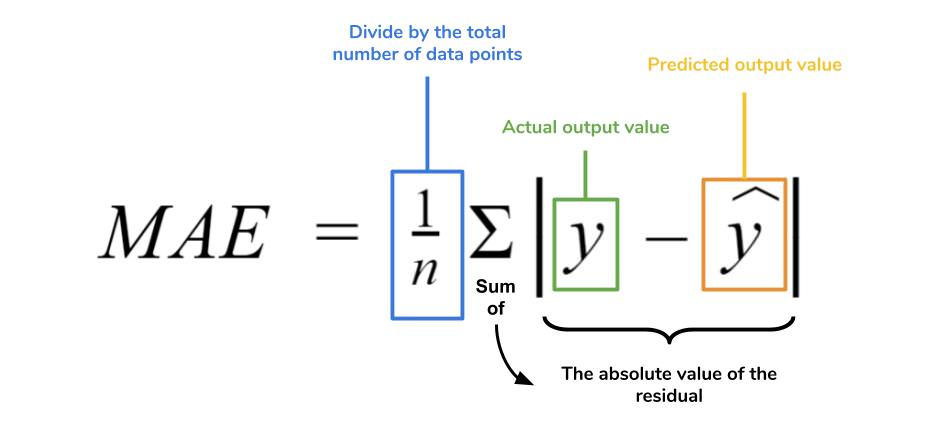top of page
Search

# Linear Regression Explained and Hands on project

Many of you have asked me when are the Machine Learning course blogs getting started. No waiting now! The first algorithm of machine learning is here for you.

The most distinguishing feature of this blog is, I am going to make Linear Regression(basic and boring topic) very interesting. How am I going to do this?

Yes, you read the title, with a very interesting use-case project which we will solve together with linear regression.

If you don't know in which of the three categories Linear Regression falls upon, check this link HERE. It will give you more insights into the different categories of machine learning.

Let's Get Started ML community

Linear regression consist of 2 variables:Linear regression can be seen as a line in X and Y coordinates which can be used to define the relationship between the dependent variable(y) and independent variables(x)Anything popped-up in your mind? Yes, it's the equation of a line.

A more machine learning way of writing a line's equation is given here:-The linear model makes a prediction by simply computing a weighted sum of the input features, plus a constant called the bias term (also called the intercept term), as shown in the equation above.

Now the question arises, How to calculate weights(theta) or y-intercept to make the line best fits the data points.The answer is the first set of weights(thetas) are randomly assigned. Then there are different metrics by which we track the progress of the weights. Those metrics can be called Evaluation metrics.

Because of the learning purpose, I will be using 3 metrics to evaluate the linear regression in the code.

The 3 metrics used are:

• Mean Absolute Error (MAE)

• Mean Square Error (MSE)

• Root Mean Square Error (RSME)

Let's get into the equations of the above metrics.

1. Mean Absolute Error (MAE)2. Mean Square Error (MSE)3. Root Means Square Error (RMSE)Taking a simple example for more insight into Linear Regression. In an e-commerce website, we have numerous features, we want to see which features are correlating with other features so that if we make some changes in one feature then we can see some profitable changes in another feature. In the practical session, you will find out that among numerous features 'Yearly Amount Spent' & 'Length of Membership' are the highly correlated features, therefore, the insight of data was, if we increase the number of people who will take membership of that e-commerce site, the yearly amount spent on that e-commerce site will linearly increase!

Alright! That's enough of theory, Let's get our hands dirty.

HERE IS THE CODE

PS: Yes, You have to open a Kaggle account. If you didn't have it yet, it's a HIGH TIME you must have.

Credits: the image source is from Dataquest and towardsdatascience.